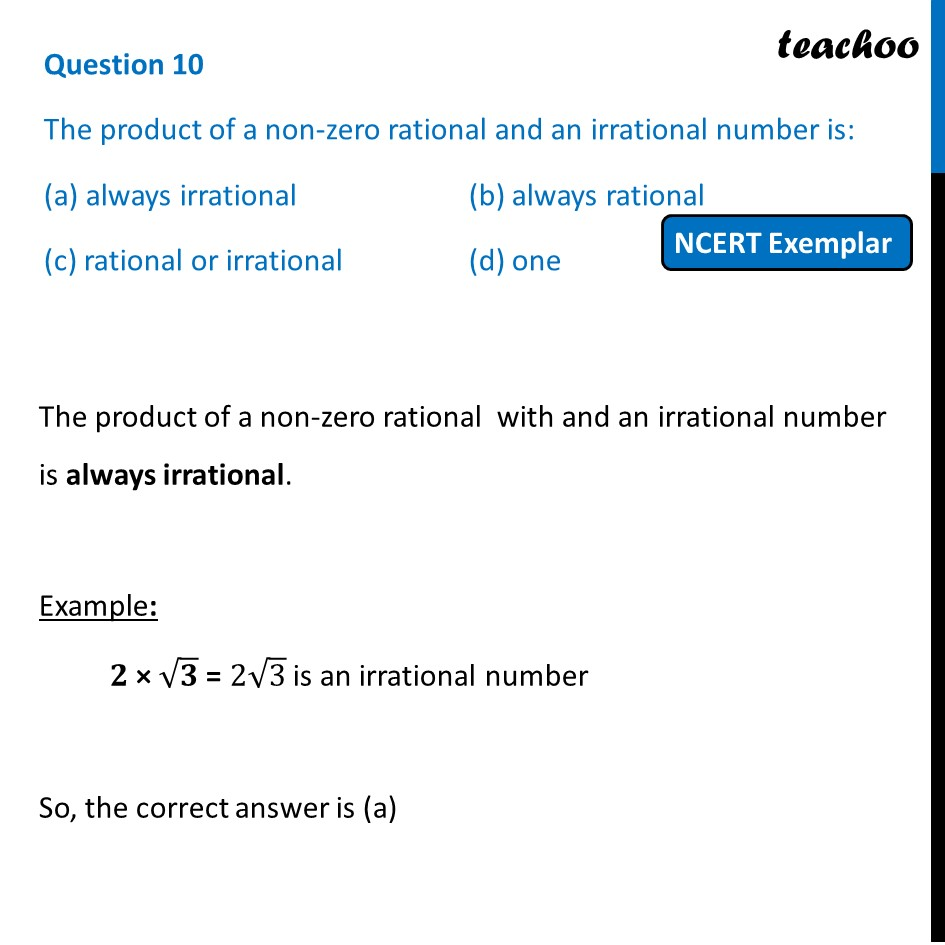## (c) rational or irrational             (d) one1. Chapter 1 Class 10 Real Numbers (Term 1)
2. Serial order wise
3. MCQs from NCERT Exemplar

Transcript

Question 10 The product of a non-zero rational and an irrational number is: (a) always irrational (b) always rational (c) rational or irrational (d) one The product of a non-zero rational with and an irrational number is always irrational. Example: 𝟐 × √𝟑 = 2√3 is an irrational number So, the correct answer is (a)

MCQs from NCERT Exemplar